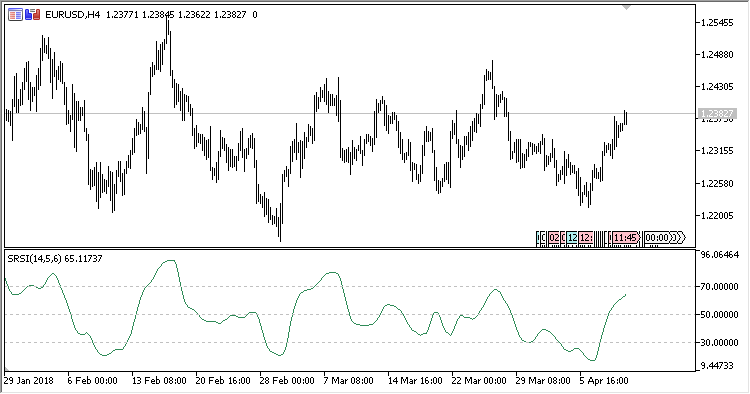Interesting script?
So post a link to it -
let others appraise it

You liked the script? Try it in the MetaTrader 5 terminal# RSI_With_Step_MA - indicator for MetaTrader 5

Views:
1292
Rating:
Published:
2018.06.06 14:32

RSI calculated using the specified smoothing type is displayed in a separate window.

It has five input parameters:

• RSI period - RSI calculation period;
• Step - the range for calculating the positive and the negative components of RSI;
• MA period - smoothing period;
• MA method - smoothing method;
• MA applied price - price used for the calculation of the smoothing MA.

Calculation:

RSI[i] = 100-(100/(1 + Positive/Negative))

where

```Positive, Negative - the sum of positive and negative increments (Diff),
Diff[i] = MA(Period_MA)[i]-MA(Period_MA)[i-Step]
```Translated from Russian by MetaQuotes Software Corp.
Original code: https://www.mql5.com/ru/code/20451Repulse_Divergence

Divergences of the Repulse oscillator.Repulse

An indicator of possible price "rebounds".Trend_Force

An indicator of trend strength.Futures Portfolio Control Expiration

A portfolio EA for the MOEX FORTS market.